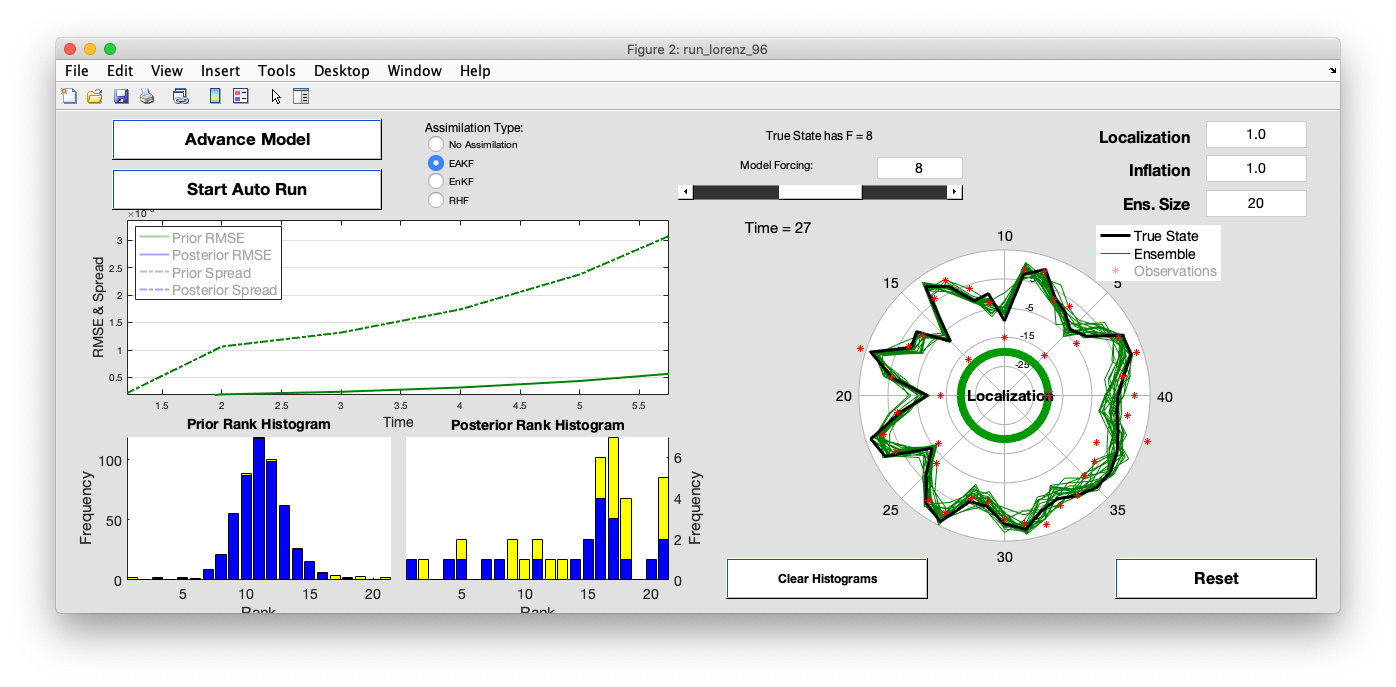January 24, 2020 by dart

## DART LAB#### An introduction to Data Assimilation using MATLAB

DART_LAB is a MATLAB®-based tutorial to demonstrate the principles of ensemble data assimilation. The DART_LAB tutorial begins at a more introductory level than the materials in the tutorial directory, and includes hands-on exercises. DART_LAB consists of PDF tutorial materials and MATLAB® exercises. See below for links to the PDF files and a list of the corresponding MATLAB scripts.

#### DART_LAB tutorial slides

1. The basics in 1D.

2. Multivariate assimilation. How should observations of a state variable impact an unobserved state variable?

3. Sampling error and localization.

4. The Ensemble Kalman Filter (Perturbed Observations).

#### Hands-on Exercises

The DART_LAB materials are bundled with DART.

``````git clone https://github.com/NCAR/DART.git
``````

In the `guide/DART_LAB/matlab` subdirectory are a set of MATLAB scripts and graphical user interface (GUI) programs which are exercises that go with the tutorial. Each is interactive with settings that can be changed and rerun to explore various options. A valid MATLAB license is needed to run these scripts.

The exercises use the following functions:

functiondescription
`gaussian_product`graphical representation of the product of two gaussians
`oned_ensemble`explore the details of ensemble data assimilation for a scalar
`oned_model`simple ensemble data assimilation example
`oned_model_inf`simple ensemble data assimilation example with inflation
`run_lorenz_63`ensemble DA with the 3-variable Lorenz ‘63 dynamical model - the “butterfly” model
`run_lorenz_96`ensemble DA with the 40-variable Lorenz ‘96 dynamical model
`run_lorenz_96_inf`ensemble DA with the 40-variable Lorenz ‘96 dynamical model with inflation
`twod_ensemble`demonstrates the impact of observations on unobserved state variables